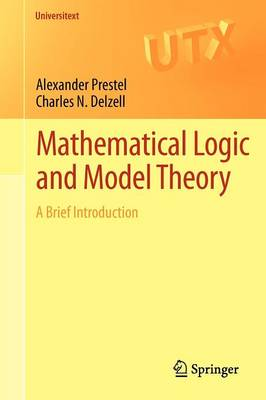•# Mathematical Logic and Model Theory - Universitext (Paperback)

(author), (author)
£29.99
Paperback 204 Pages / Published: 21/08/2011
• We can order this from the publisher

UK delivery within 3-4 weeks

Mathematical Logic and Model Theory: A Brief Introduction offers a streamlined yet easy-to-read introduction to mathematical logic and basic model theory. It presents, in a self-contained manner, the essential aspects of model theory needed to understand model theoretic algebra. As a profound application of model theory in algebra, the last part of this book develops a complete proof of Ax and Kochen's work on Artin's conjecture about Diophantine properties of p-adic number fields. The character of model theoretic constructions and results differ quite significantly from that commonly found in algebra, by the treatment of formulae as mathematical objects. It is therefore indispensable to first become familiar with the problems and methods of mathematical logic. Therefore, the text is divided into three parts: an introduction into mathematical logic (Chapter 1), model theory (Chapters 2 and 3), and the model theoretic treatment of several algebraic theories (Chapter 4). This book will be of interest to both advanced undergraduate and graduate students studying model theory and its applications to algebra. It may also be used for self-study.

Publisher: Springer London Ltd
ISBN: 9781447121756
Number of pages: 204
Weight: 320 g
Dimensions: 234 x 156 x 10 mm
Edition: 2011